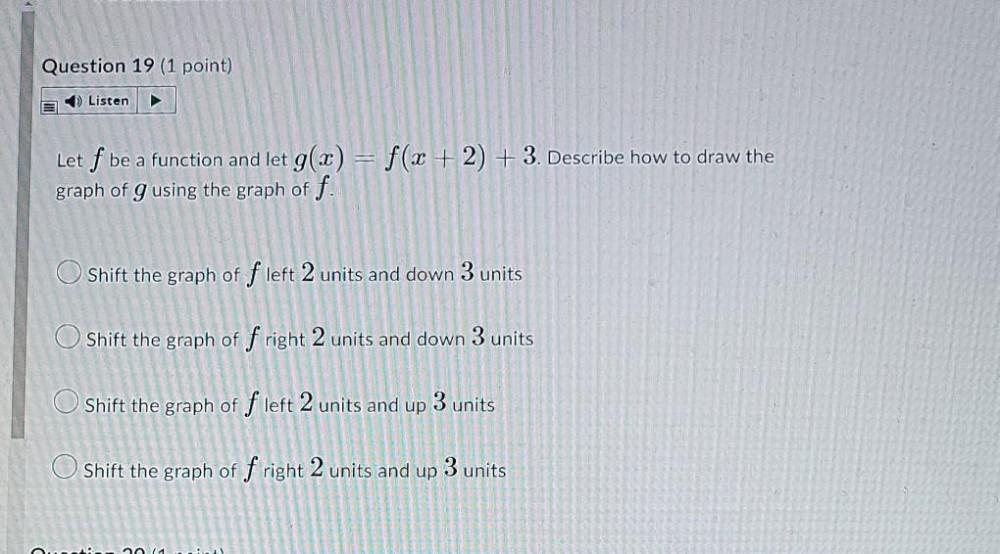Question:

# Let f be a function and let g(x) = f(x + 2) + 3 . Describe how to draw the graph of g using the graph of f. ○Shift the graph of f left 2 units and down 3 units ○Shift the graph of f right 2 units andLet f be a function and let g(x) = f(x + 2) + 3 . Describe how to draw the graph of g using the graph of f. ○Shift the graph of f left 2 units and down 3 units ○Shift the graph of f right 2 units and down 3 units ○Shift the graph of f left 2 units and up 3 units ○Shift the graph of f right 2 units and up 3 units